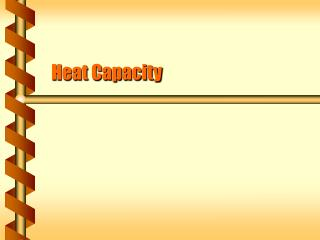DownloadDownload PresentationHeat Capacity

# Heat Capacity

Télécharger la présentation## Heat Capacity

- - - - - - - - - - - - - - - - - - - - - - - - - - - E N D - - - - - - - - - - - - - - - - - - - - - - - - - - -
##### Presentation Transcript

1. Heat Capacity

2. The work - energy principle applies to all objects. If an object speeds up it gains kinetic energy. If an object slows down it loses kinetic energy. Work In Work done on a system v1 v2

3. Temperature change is due to heat (Q). If an object’s temperature increases it gains heat. If an object’s temperature decreases it loses heat. Heat In Heat into a system

4. Heat Capacity • The ratio of the change in heat to the change in temperature is the heat capacity (C). • Depends on material • Depends on the mass • Measured in J/K

5. The ratio of the change in heat to the change in temperature is the heat capacity (C). Depends on material independent of the mass Measured in J/kg-K MaterialSpecific heat Mercury 140 J/kg-K Copper 390 J/kg-K Steel 500 J/kg-K Granite 840 J/kg-K Aluminum 900 J/kg-K Wood 1400 J/kg-K Ice 2100 J/kg-K Water 4200 J/kg-K Specific Heat

6. You are last to use the shower today and the temperature in the water heater has dropped to 18 C from the normal temperature of 50 C. The heater holds 150 kg and has a 50 kW heating coil. How long should you wait before starting your shower? Find the heat required. DQ = mcDT = (150 kg) (4200 J/kg-K) ( 32 C) DQ = 2.1 x 107 J Find the time from the power, and set the heat to the work. P = DW/Dt t = DQ / P (2.1 x 107 J) / (5 x 104 W) t = 4000 s = 1.1 hr Take a Shower

7. Equilibrium Temperature • Two systems in thermal contact will adjust to reach the same temperature - thermal equilibrium • If they are thermally insulated, all the heat goes from the hot system to the cold system. 1 2

8. A 1.5 kg aluminum pan is heated to 180 C then placed into a sink with 8 kg water at 20 C. If no water boils, what is the equilibrium temperature? Setup the equation for an unknown equilibrium temperature T. Solve for T. T = 26 C Doing Dishes next